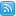# W3C Math Home

## What is MathML?

MathML is a low-level specification for mathematical and scientific content on the Web and beyond.

These pages provide you with information on how to learn and use MathML, on available software, and news from the MathML community.

Here is an example of Presentation MathML: $\left(\begin{array}{cc}a& b\\ c& d\end{array}\right)\left(\begin{array}{c}x\\ y\end{array}\right)=\left(\begin{array}{c}e\\ f\end{array}\right)$ and another: $\left\{\begin{array}{c}ax+by=e\\ cx+dy=f\end{array}$

### High­lights(Images: whiteboard by Jeswin Thomas

## Specs&Drafts

Com­pleted Current Next
MathML 3 REC
XML Entity Definitions REC
MathML 2 REC
Units in MathML NOTE
Arabic mathematical notation NOTE
Structured Types in MathML 2.0 NOTE
Bound Variables in MathML NOTE
Revising Current Next
MathML Core WD WD
MathML 4 FPWD WD
MathML Media-type Declarations Note Note
Unmaintained Current Next
A MathML for CSS Profile REC

## LearnWhat is MathML teaches you how to create your first equation and to understand the base constructs of MathML.

For a quick intro, try Mozilla's tutorial, Daniel Scully's Beginner's Guide, or the Connexions Guide to MathML.

For a thorough resource on all tags and attributes, dive into the excellent documentation at Mozilla and you can also simply check out some pretty examples of MathML.

## SoftwareAll major browsers support MathML and support in math & science applications is ubiquitous. To write MathML, you don't need more than a text editor, but there are many tools available that make it even easier. The MathWG keeps a non-exhaustive list on its public wiki, including browser support, JavaScript polyfills, authoring&conversion tools and more general MathML-enabled software.

Of course, nearly all software has bugs. And some programs are further ahead implementing MathML 3 than others.

## Joining the discussion

The Math Working Group discusses on the <www-math@w3.org> mailing list. Everybody can subscribe (or unsubscribe, or see instructions.)

You can also read and create issues on GitHub. (You need a (free) GitHub account to create a new issue.)

The ‘MathML Refresh’ Community Group developed drafts for MathML 4 and MathML Core, preparing for the Working Group. Anybody can join the group.

## The Math Working Group

The Math Working Group is one of the oldest W3C Working Groups. During its first period of activity (1996–2004), it created and maintained versions 1 and 2 of MathML. During its second period (2006–2016), it created, among other things, MathML version 3 and saw it become an ISO standard.

Since April 2021, the group is developing a new revision of MathML, MathML version 4, as well as MathML Core, a subset that can be reliably displayed in web browsers.Bert Bos, math activity lead
Last modified: $Date: 2023/05/15 15:49:00$ UTC